# Number theory

(diff) ← Older revision | Latest revision (diff) | Newer revision → (diff)

The science of integers (cf. also Integer). Integers, together with the simplest geometrical figures, were the first and the most ancient mathematical concepts. Number theory arose from problems in arithmetic connected with the multiplication and division of integers.

In Ancient Greece (6th century B.C.) divisibility of integers was studied, and particular subclasses of integers (such as prime numbers, cf. Prime number, composite numbers, squares) were distinguished; the structure of perfect numbers (cf. Perfect number) was studied; and the solution in integers of the equationwas given, that is, an algorithm was described to construct right-angled triangles with sides of integer lengths. Euclid (3rd century B.C.) in his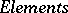gave a systematic development of the theory of divisibility, based on the so-called Euclidean algorithm for finding the greatest common divisor of two integers, and proved the first theorem in the theory of prime numbers: There are infinitely many prime numbers. Somewhat later, Eratosthenes discovered a method of obtaining prime numbers, which is still called the sieve of Eratosthenes (cf. Eratosthenes, sieve of).

A systematization of the problems in number theory, and methods for their solution, was carried out by Diophantus (3rd century) in his Aritmetika, where, in particular, he gave solutions in rational numbers of many algebraic equations of the first and second degrees in several unknowns.

In China, from the 2nd century onwards, in connection with calendar computations, the problem arose of finding the least integer that yields given remainders on division by given numbers. This was solved by Sun-tsi (2nd–6th centuries) and by Tsin Tsiushao (13th century) (cf. Chinese remainder theorem).

In India, Bramagupta (7th century) and Bhaskara (12th century) gave general methods for solving in integers indefinite equations of the first degree in two unknowns, as well as of equations of the form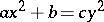and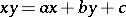.

The golden age of number theory in Europe began with the work of P. Fermat (17th century). He investigated the solutions of many equations in integers, and in particular conjectured that the equation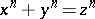,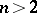, has no solution in natural numbers(Fermat great theorem); he proved that a prime number of the form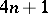is a sum of two squares, and asserted one of the basic results of the theory of congruences: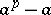is divisible byifis a prime number (Fermat little theorem).

L. Euler made an exceptionally important contribution to number theory. He proved Fermat's great theorem for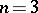, Fermat's little theorem and a generalization of it, and a whole series of theorems about representations of numbers by binary quadratic forms (cf. Binary quadratic form). Euler was the first to approach solutions to problems in number theory by means of mathematical analysis, which led to the creation of analytic number theory. Euler's generating functions (cf. Generating function) appeared as the source of the circle method of Hardy–Littlewood–Ramanujan and the method of trigonometric sums (cf. Trigonometric sums, method of) of I.M. Vinogradov — the basic methods of modern additive number theory, while the function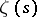, introduced by Euler, and its generalizations, are the basis of the contemporary analytic methods used to investigate the problem of the distribution of prime numbers.

In the correspondence between Ch. Goldbach and Euler, three famous problems were posed: Whether every odd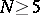is a sum of three primes; whether every even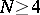is a sum of two primes; and whether every oddhas the form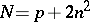, whereis a prime number andan integer. The first problem was solved by Vinogradov (1937), and the other two remain unsolved (1989).

C.F. Gauss created the basic methods in and completed the construction of the theory of congruences (cf. Congruence), proved the quadratic reciprocity law (cf. also Gauss reciprocity law; Reciprocity laws), which had been formulated by Euler, laid the foundations of the theory of representations of numbers by quadratic formsand by forms of higher degrees in several variables, introduced the so-called Gauss sums (cf. Gauss sum)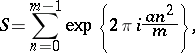which were the first trigonometric sums, and showed that they were useful in solving problems in number theory. While number theory before the time of Gauss had been merely a collection of different results and ideas, after his work it began to develop in several directions as a harmonized theory.

Great contributions to number theory have been made by many scholars in the 19th century and 20th century.

One of the peculiarities and attractions of number theory is the simplicity and accessibility of the majority of its problems, and the difficulty of solving them. For example, the problem of whether there are infinitely many prime twins was posed by Euclid and is still unsolved (1989). Individual problems in number theory have become sources of important independent branches of mathematics. Among these are: the theory of prime numbers (cf. Prime number) and the related theory of the zeta-function and Dirichlet series, the theory of Diophantine equations; additive number theory, the metric theory of numbers, the theory of algebraic and transcendental numbers, algebraic number theory, the theory of Diophantine approximations, probabilistic number theory (cf. Number theory, probabilistic methods in), and the geometry of numbers. For example, a source of analytic number theory was the problem of the distribution of primes in series of natural numbers and the problem of representing natural numbers as sums of terms of a particular form. Solving equations in integers, in particular Fermat's great theorem, was the source of algebraic number theory. The problem of constructing a disc of unit area by means of a straightedge and a compass (cf. Quadrature of the circle) led to questions about the arithmetic nature of the number, and hence to the creation of the theory of algebraic and transcendental numbers.

All the above branches of number theory are interconnected, complementing and enriching one another.

How to Cite This Entry:
Number theory. Encyclopedia of Mathematics. URL: http://encyclopediaofmath.org/index.php?title=Number_theory&oldid=14109
This article was adapted from an original article by A.A. KaratsubaMaterial from the article "Number theory" in BSE-2 (originator), which appeared in Encyclopedia of Mathematics - ISBN 1402006098. See original article